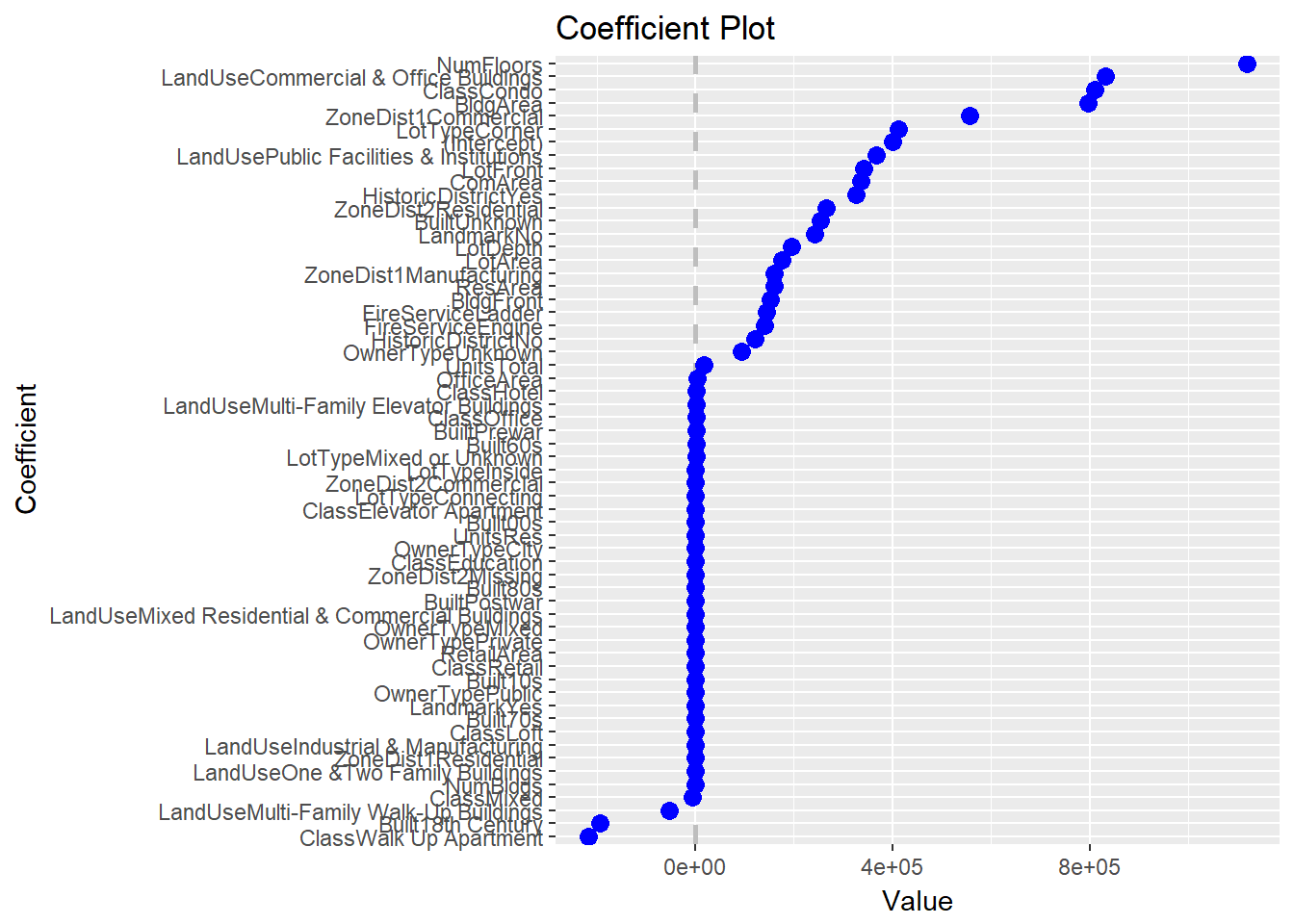In my last post I discussed using `coefplot` on `glmnet` models and in particular discussed a brand new function, `coefpath`, that uses `dygraphs` to make an interactive visualization of the coefficient path.

Another new capability for version 1.2.5 of `coefplot` is the ability to show coefficient plots from `xgboost` models. Beyond fitting boosted trees and boosted forests, `xgboost` can also fit a boosted Elastic Net. This makes it a nice alternative to `glmnet` even though it might not have some of the same user niceties.

To illustrate, we use the same data as our previous post.

First, we load the packages we need and note the version numbers.

``````# list the packages that we load
# alphabetically for reproducibility
packages <- c('caret', 'coefplot', 'DT', 'xgboost')
# call library on each package
purrr::walk(packages, library, character.only=TRUE)

# they are listed here for complete documentation
packagesColon <- c('dplyr', 'dygraphs', 'knitr', 'magrittr', 'purrr', 'tibble', 'useful')``````
``````versions <- c(packages, packagesColon) %>%
purrr::map(packageVersion) %>%
purrr::map_chr(as.character)
packageDF <- tibble::data_frame(Package=c(packages, packagesColon), Version=versions) %>%
dplyr::arrange(Package)
knitr::kable(packageDF)``````
Package Version
caret 6.0.78
coefplot 1.2.6
dplyr 0.7.4
DT 0.2
dygraphs 1.1.1.4
knitr 1.18
magrittr 1.5
purrr 0.2.4
tibble 1.4.2
useful 1.2.3
xgboost 0.6.4

Then, we read the data. The data are available at https://www.jaredlander.com/data/manhattan_Train.rds with the CSV version at data.world. We also get validation data which is helpful when fitting `xgboost` mdoels.

``````manTrain <- readRDS(url('https://www.jaredlander.com/data/manhattan_Train.rds'))

The data are about New York City land value and have many columns. A sample of the data follows. There’s an odd bug where you have to click on one of the column names for the data to display the actual data.

``````datatable(manTrain %>% dplyr::sample_n(size=1000), elementId='TrainingSampled',
rownames=FALSE,
options=list(
scroller=TRUE
))``````

While `glmnet` automatically standardizes the input data, `xgboost` does not, so we calculate that manually. We use `preprocess` from `caret` to compute the mean and standard deviation of each numeric column then use these later.

``preProc <- preProcess(manTrain, method=c('center', 'scale'))``

Just like with `glmnet`, we need to convert our `tbl` into an X (predictor) `matrix` and a Y (response) `vector`. Since we don’t have to worry about multicolinearity with `xgboost` we do not want to drop the baselines of `factor`s. We also take advantage of sparse matrices since that reduces memory usage and compute, even though this dataset is not that large.

In order to build the `matrix` and `vector` we need a `formula`. This could be built programmatically, but we can just build it ourselves. The response is `TotalValue`.

``````valueFormula <- TotalValue ~ FireService + ZoneDist1 + ZoneDist2 +
Class + LandUse + OwnerType + LotArea + BldgArea + ComArea + ResArea +
OfficeArea + RetailArea + NumBldgs + NumFloors + UnitsRes + UnitsTotal +
LotDepth + LotFront + BldgFront + LotType + HistoricDistrict + Built +
Landmark``````
``````manX <- useful::build.x(valueFormula, data=predict(preProc, manTrain),
# do not drop the baselines of factors
contrasts=FALSE,
# use a sparse matrix
sparse=TRUE)

manY <- useful::build.y(valueFormula, data=manTrain)

manX_val <- useful::build.x(valueFormula, data=predict(preProc, manVal),
# do not drop the baselines of factors
contrasts=FALSE,
# use a sparse matrix
sparse=TRUE)

manY_val <- useful::build.y(valueFormula, data=manVal)``````

There are two functions we can use to fit `xgboost` models, the eponymous `xgboost` and `xgb.train`. When using `xgb.train` we first store our X and Y matrices in a special `xgb.DMatrix` object. This is not a necessary step, but makes things a bit cleaner.

``````manXG <- xgb.DMatrix(data=manX, label=manY)
manXG_val <- xgb.DMatrix(data=manX_val, label=manY_val)``````

We are now ready to fit a model. All we need to do to fit a linear model instead of a tree is set `booster='gblinear'` and `objective='reg:linear'`.

``````mod1 <- xgb.train(
# the X and Y training data
data=manXG,
# use a linear model
booster='gblinear',
# minimize the a regression criterion
objective='reg:linear',
# use MAE as a measure of quality
eval_metric=c('mae'),
# boost for up to 500 rounds
nrounds=500,
# print out the eval_metric for both the train and validation data
watchlist=list(train=manXG, validate=manXG_val),
# print eval_metric every 10 rounds
print_every_n=10,
# if the validate eval_metric hasn't improved by this many rounds, stop early
early_stopping_rounds=25,
# penalty terms for the L2 portion of the Elastic Net
lambda=10, lambda_bias=10,
# penalty term for the L1 portion of the Elastic Net
alpha=900000000,
# randomly sample rows
subsample=0.8,
# randomly sample columns
col_subsample=0.7,
# set the learning rate for gradient descent
eta=0.1
)``````
``````##   train-mae:1190145.875000    validate-mae:1433464.750000
## Multiple eval metrics are present. Will use validate_mae for early stopping.
## Will train until validate_mae hasn't improved in 25 rounds.
##
##  train-mae:938069.937500 validate-mae:1257632.000000
##  train-mae:932016.625000 validate-mae:1113554.625000
##  train-mae:931483.500000 validate-mae:1062618.250000
##  train-mae:931146.750000 validate-mae:1054833.625000
##  train-mae:930707.312500 validate-mae:1062881.375000
##  train-mae:930137.375000 validate-mae:1077038.875000
## Stopping. Best iteration:
##  train-mae:931146.750000 validate-mae:1054833.625000``````

The best fit was arrived at after 41 rounds. We can see how the model did on the train and validate sets using `dygraphs`.

``dygraphs::dygraph(mod1\$evaluation_log)``

We can now plot the coefficients using `coefplot`. Since `xgboost` does not save column names, we specify it with `feature_names=colnames(manX)`. Unlike with `glmnet` models, there is only one penalty so we do not need to specify a specific penalty to plot.

``coefplot(mod1, feature_names=colnames(manX), sort='magnitude')``This is another nice addition to `coefplot` utilizing the power of `xgboost`.

#### Related PostsJared Lander is the Chief Data Scientist of Lander Analytics a New York data science firm, Adjunct Professor at Columbia University, Organizer of the New York Open Statistical Programming meetup and the New York and Washington DC R Conferences and author of R for Everyone.